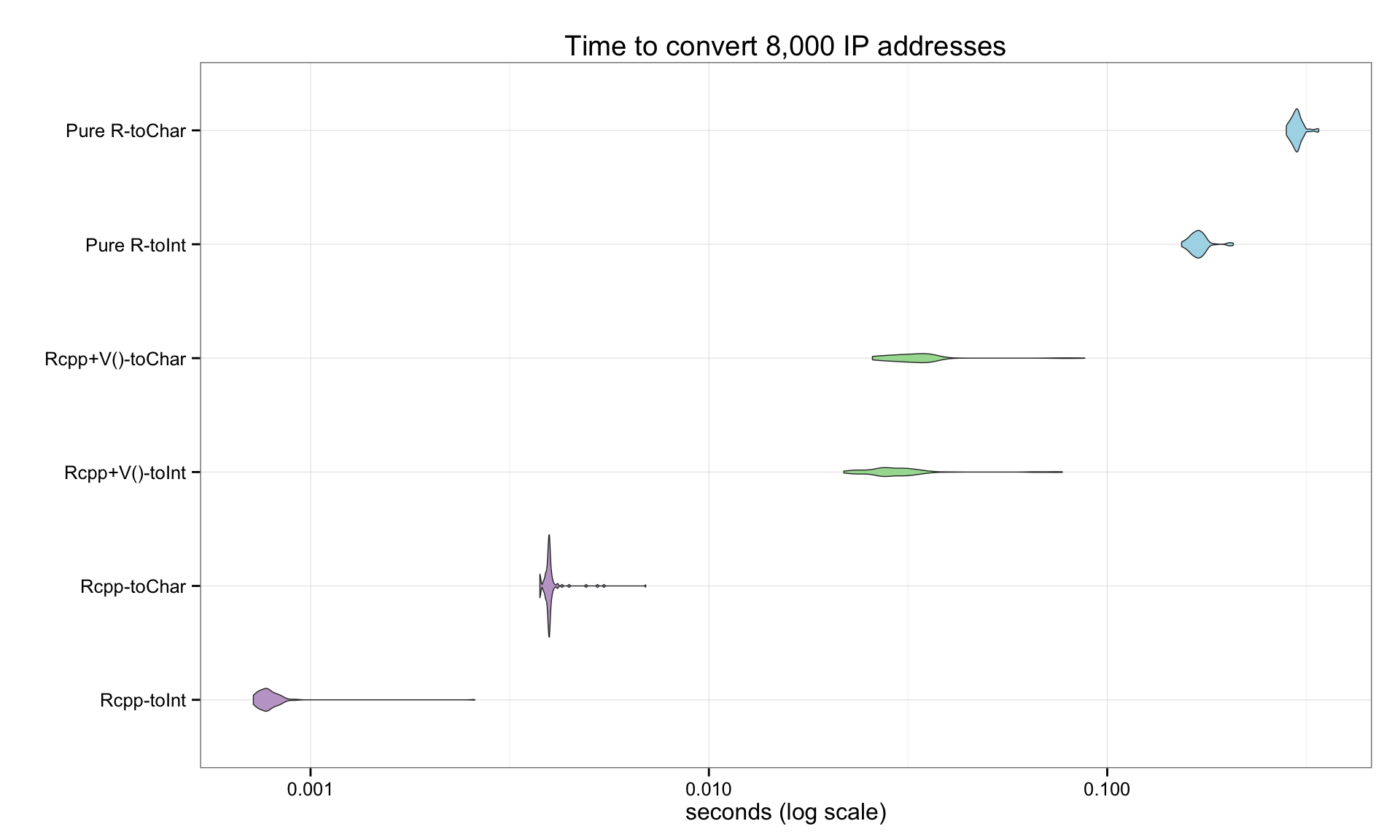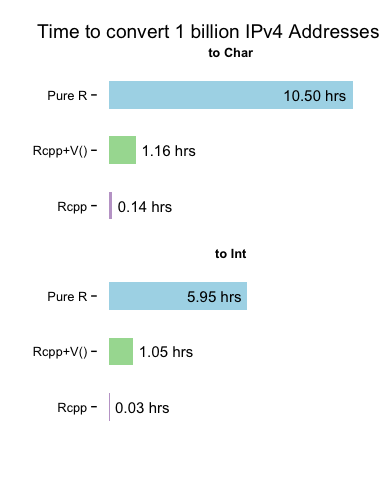Vectorizing IPv4 Address Conversions - Part 2

By Bob Rudis (@hrbrmstr)
Sat 17 May 2014 | tags: rstats, r, rcpp, -- (permalink)

The previous post looked at using the `Vectorize()` function to, well, vectorize, our Rcpp IPv4 functions. While this is a completely acceptable practice, we can perform the vectorization 100% in `Rcpp`/C++. We’ve included both the original `Rcpp` IPv4 functions and the new `Rcpp`-vectorized functions together to show the minimal differences between them:

```#include <Rcpp.h>

using namespace Rcpp;
using namespace boost::asio::ip;

// Rcpp/C++ vectorized routines

// [[Rcpp::export]]
NumericVector rcpp_rinet_pton (CharacterVector ip) {

int ipCt = ip.size(); // how many elements in vector

NumericVector ipInt(ipCt); // allocate new numeric vector

// CONVERT ALL THE THINGS!
for (int i=0; i<ipCt; i++) {
}

return(ipInt);
}

// [[Rcpp::export]]
CharacterVector rcpp_rinet_ntop (NumericVector ip) {

int ipCt = ip.size();

CharacterVector ipStr(ipCt); // allocate new character vector
// CONVERT ALL THE THINGS!
for (int i=0; i<ipCt; i++) {
}

return(ipStr);

}

// orignial single-element vector routines we'll vectorize with Vectorize()

// [[Rcpp::export]]
unsigned long rinet_pton (CharacterVector ip) {
}

// [[Rcpp::export]]
CharacterVector rinet_ntop (unsigned long addr) {
}
```

We’ve merely wrapped a `for` loop around the original code and built the result vectors in `Rcpp`, relying on the object-oriented nature of C++ for proper value conversion+assignment. The pure-R+`Vectorize()`‘d code (from the examples in the book) is below, since we’re going to pit all three in a head-to-head performance competition.

```# Vectorize() the single-element vector routines
v_rinet_pton <- Vectorize(rinet_pton, USE.NAMES=FALSE)
v_rinet_ntop <- Vectorize(rinet_ntop, USE.NAMES=FALSE)

# pure R version with Vectorize()
ip2long <- Vectorize(function(ip) {
ips <- unlist(strsplit(ip, '.', fixed=TRUE))
octet <- function(x,y) bitOr(bitShiftL(x, 8), y)
Reduce(octet, as.integer(ips))
}, USE.NAMES=FALSE)

long2ip <- Vectorize(function(longip) {
octet <- function(nbits) bitAnd(bitShiftR(longip, nbits), 0xFF)
paste(Map(octet, c(24,16,8,0)), sep="", collapse=".")
}, USE.NAMES=FALSE)
```

Now, we’ll read in a file of ~8,000 IPv4 addresses, make them into integers and then use the `microbenchmark` package to profile the to/from conversion of all three versions of the routines.

```# read in ~8K IP address strings & make ints for our benchmark
ints <- rcpp_rinet_pton(ips\$V1)

# run a benchmark 100 times per routine, giving plenty of "ramp up" time
mb <- microbenchmark(rcpp_ints <- rcpp_rinet_pton(ips\$V1),
rcpp_chars <- rcpp_rinet_ntop(ints),
v_ints <- v_rinet_pton(ips\$V1),
v_chars <- v_rinet_ntop(ints),
r_ints <- ip2long(ips\$V1),
r_chars <- long2ip(ints),
control=list(warmup=20),
times=100, unit="s")
```

Then, we’ll take a look at the results (all times are in seconds):

Version min lq median uq max
Rcpp-toInt 0.0007216090 0.0007610835 0.0007967235 0.0008572075 0.0026142800
Rcpp-toChar 0.0037574850 0.0038886490 0.0039565840 0.0040140285 0.0046188840
Rcpp+V()-toInt 0.0217142230 0.0266931380 0.0290988580 0.0316722610 0.0775550730
Rcpp+V()-toChar 0.0253528670 0.0290143845 0.0322646160 0.0346684450 0.0814177860
Pure R-toInt 0.1480684080 0.1588533500 0.1654142360 0.1701886530 0.1992565150
Pure R-toChar 0.2726176440 0.2863672665 0.2917557870 0.2960467515 0.3371749450

If we just look at the median values, we can see that the conversion to integer takes:

Version median
Rcpp-toInt 0.0007967235
Rcpp+V()-toInt 0.0290988580
Pure R-toInt 0.1654142360

and, the conversion to character takes:

Version median
Rcpp-toChar 0.0039565840
Rcpp+V()-toChar 0.0322646160
Pure R-toChar 0.2917557870

But, a visualization is (often) worth a dozen tables, so we’ll take the test results and make a violin plot (which is just a more granular boxplot). Note that the plot is on a log scale, so the differences between each set of comparisons are actually much larger than your eye will initially comprehend (hence the inclusion of the above tables).It’s often difficult for us to grok fractional seconds, so let’s do some basic math to see how long each method would take to process 1 billion IP addresses. We’ll use the median values from above and compare the results in a simple bar chart:The fully vectorized `Rcpp` versions are the clear “winners” and will let us scale our IPv4 address conversions to millions, billions or trillions of operations without having to rely on other scripting languages. We can use this base as foundation for a complete IP address `S4` class that we’ll cover in future posts.

You can find the `Rmd` source that helped generate this post over at github along with the data file.